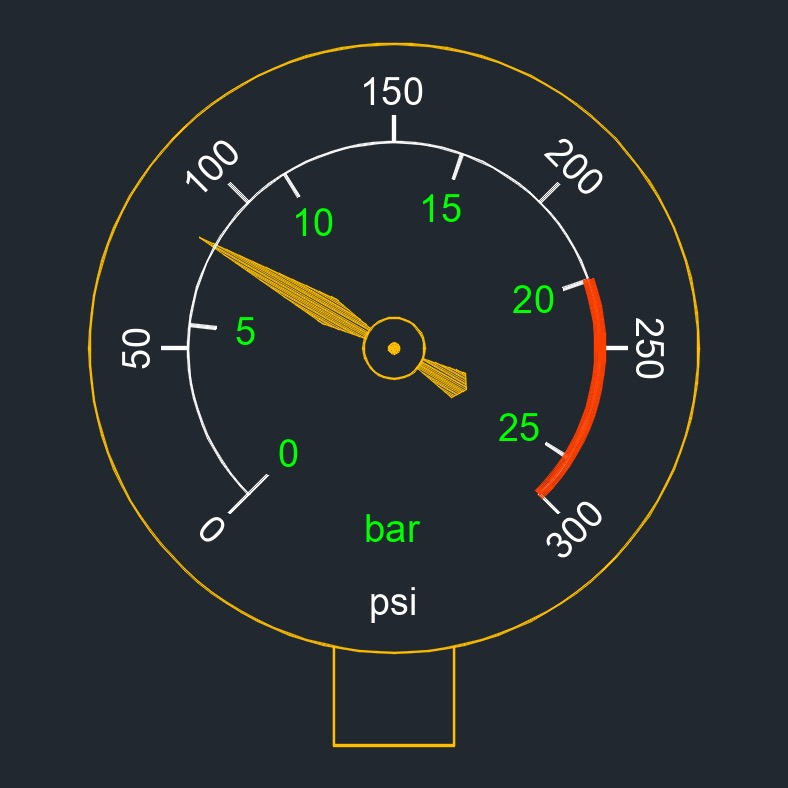# Pressure

on . Posted in Classical MechanicsPressure, abbreviated as p, is one of the most important concepts in piping design.  Pressure is a measure of the force exerted per unit area on a surface.  It is defined as the force per unit area perpendicular to the surface over which the force is distributed.

Pressure can be expressed as either absolute pressure or gauge pressure.  Absolute pressure is the total pressure measured relative to a perfect vacuum, while gauge pressure is the difference between the absolute pressure and the ambient pressure of the surrounding environment.  Pressure is a scalar quantity having magnitude and is independent of any specific direction.

Pressure plays a fundamental role in many areas of science and engineering, including fluid dynamics, thermodynamics, and materials science.  It is used to describe and predict the behavior of gases, liquids, and solids under various conditions, and is essential in the design and operation of a wide range of devices, from engines and turbines to pumps and compressors.

### Pressure Types

• Absolute Pressure  -  The pressure referenced to an absolute vacuum.
• Atmospheric Pressure  -  The pressure exerted upon the earth's surface by the air because of the gravitational attraction of the earth.
• Back Pressure  -  The pressure held back on the upstream side of a system.
• Breakout Pressure  -  The minimum pressure which starts moving an actuator.
• Cracking Pressure  -  The pressure at which the fluid starts to leak through the valve before the set pressure is reached.
• Critical Pressure  -  The highest temperature at which well defined liquid and vapor states exist.  It may be defined as the highest temperature at which it is possible to liquefy a gas by pressure alone.
• Discharge Pressure  -  The total gas pressure (static plus velocity) at the discharge flange of the compressor.  Velocity pressure usually is considered only with dynamic pressure.
• Full-flow Pressure  -  The pressure at which a valve is wide open and passes its full flow.
• Gauge Pressure  -  Measures pressure referenced to local atmospheric pressure and is vented to the atmosphere.
• Inlet Pressure  -  Pressure at the intake to the compressor.  Usually expressed as absolute pressure.
• Kick-in Pressure  -  Factory set low pressure point of the pressure switch that starts the compressor to re-pressurize the tank to a higher pressure.
• Kick-out Pressure  -  Factory set high pressure point of the pressure switch that stops the compressor from increasing the pressure in the tank above a certain level.
• Negative Pressure  -  Pressure is normally positive, but negative pressure is when the enclosed pressure is lower than the area around it.
• Operating Pressure (Working Pressure)  -  The pressure at which a system is normally operated.
• Osmotic Pressure  -  The pressure created by the difference in concentration of the constituents on either side of the membrane, and this pressure drives the osmosis process.
• Pilot Pressure  -  Auxiliary pressure used to actuate or control a component.
• Pressure Differential  -  Used to describe the amount of pressure created by a pump or compressor.  It also is used for pressure drop in a pipe, piece of equipment or an orifice plate.
• Pressure Loss  -  The difference in pressure between two points, usually caused by friction resistance in the system,
• Rated Pressure  -  The operating pressure which is recommended for a component or a system by the manufacturer.
• Relative Pressure (Overpressure)  -  The difference between the process pressure and the prevailing atmospheric pressure.
• Sealed Pressure  -  Measures pressure referenced to the prevailing atmospheric pressure hermetically sealed within the transducer.
• Set Pressure  -  The point where a pressure relief valve (PRV) is set to start opening.
• Static Pressure  -  The difference in pressure between the suction side and pressure side.  The higher the static pressure or resistance, the more energy it takes to move the object.
• Suction Pressure  -  The absolute pressure of the fluid at the inlet side of the pump.
• Surge Pressure  -  The pressure changes caused in a system from a rapidly acceleration.  The surge includes the span of the changes, from high to low.
• System Pressure  -  The pressure which overcomes the total resistances in a system.

## Pressure formula

$$\large{ p = \frac{F}{A} }$$

### Pressure - Solve for p

$$\large{ p = \frac{F}{A} }$$

 force, F area, A

### Pressure - Solve for F

$$\large{ F = p \; A }$$

 pressure, p area, A

### Pressure - Solve for A

$$\large{ A = \frac{F}{p} }$$

 force, F pressure, p

Symbol English Metric
$$\large{ p }$$ = pressure $$\large{\frac{lbf}{in^2}}$$ $$\large{Pa}$$
$$\large{ F }$$ = force acting on the surface $$\large{lbf}$$ $$\large{N}$$
$$\large{ A }$$ = area of the surface $$\large{ft^2}$$ $$\large{m^2}$$

### Typical Units

Some common units for pressure are as follows.  For a more complete list, visit the pressure conversion page

INTERNATIONAL SYSTEM OF UNITS, SI

ENGLISH UNITS

Bar Inches of Mercury
Centimeter of Mercury Inches of Water
Centimeter of Water Kip per Foot2, KSF
Pascals, Pa Kip per Inch2, KSI
Pound per Foot2, PSF
Pound per Inch2, PSI

### Pressure Instruments

In piping design, pressure is measured several different ways.  On a Piping & Instrumentation Diagram, the typical instruments are:

• Pressure Indicator  -  It is a mechanical device, that is calibrated to display a pressure.
• Pressure Transmitter  -  This is used to display the pressure in the equipment and send an analog signal to a computer for futher processing.  It might be used as an alarm in case the pressure gets outside normal operating conditions.
• Pressure Switch  -  Used to send a digital signal (yes or no, 1 or 0) to a computer for an action to be performed.  E.g. send an alarm, turn off a pump, etc.